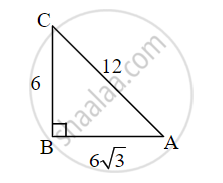# Choose the correct alternative: In ∆ABC, AB = 63 cm, AC = 12 cm, and BC = 6 cm, then m∠A = ? - Geometry

MCQ

Choose the correct alternative:

In ∆ABC, AB = 6sqrt(3) cm, AC = 12 cm, and BC = 6 cm, then m∠A = ?

• 30°

• 60°

• 90°

• 45°

#### Solution

30°We know that, 6 = 1/2(12) and

6sqrt3 = sqrt3/2 (12)

∴ BC = 1/2 "AC and AB" = sqrt3/2 "AC"

∴ ∠A = 30°   ...(Converse of 30°-60°-90° theorem)

Concept: Converse of Pythagoras Theorem
Is there an error in this question or solution?

Share